# Photocopier

A photocopier enlarges a picture in the ratio 7:4. How many times will a picture of size 6cm by 4cm be enlarged to fit on a 30cm by 20 cm page?

Result

n =  2.876

#### Solution:Leave us a comment of example and its solution (i.e. if it is still somewhat unclear...):

Showing 0 comments:Be the first to comment!#### To solve this example are needed these knowledge from mathematics:

Do you want to convert length units?

## Next similar examples:

1. Cows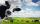4 cows spent 16 bags of hay in 5 days. How many bags of hay sacks is needed for 5 cows for seven days?
2. Numbers at ratio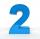The two numbers are in a ratio 3:2. If we each increase by 5 would be at a ratio of 4:3. What is the sum of original numbers?
3. Gain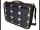Find the gain whenever the output power is 21x the input power.
4. Map 3Map scale is M = 1: 25000 . Two cottages which are shown on the map are actually 15 km away. What is its distance on the map?
5. Unknown amount of money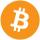Damian and Denis split an unknown amount in the ratio of 5:4 . Damian got six euros more than Denis. Calculate an unknown amount. Determine how much money got Damian and how Denis.
6. Steps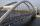Patrick step is 65 cm long and his dad step is 10 cm longer. Dad goes bridge in 52 steps. How many steps will go the same bridge by Patrick?
7. TeamsHow many ways can divide 16 players into two teams of 8 member?
8. Rectangle AntonDifference between length and width of the rectangle is 8. Length is 3-times larger than the width. Calculate the dimensions of the rectangle.
9. Theorem proveWe want to prove the sentense: If the natural number n is divisible by six, then n is divisible by three. From what assumption we started?
10. The city 3The city has 22,000 residents. How long it is expected to have 25,000 residents if the average annual population growth is 1.4%?
11. Logif ?, what is b?
12. Radioactivity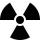After 548 hours decreases the activity of a radioactive substance to 1/9 of the initial value. What is the half-life of the substance?
13. Exponential equationIn the set R solve the equation: ?
14. LineIt is true that the lines that do not intersect are parallel?
15. AverageIf the average(arithmetic mean) of three numbers x,y,z is 50. What is the average of there numbers (3x +10), (3y +10), (3z+10) ?
16. Reference angleFind the reference angle of each angle:
17. TrigonometryIs true equality? ?